This book is archived and will be removed July 6, 2022. Please use the updated version.

Sources of Magnetic Fields

# 78 Magnetic Field Due to a Thin Straight Wire

### Learning Objectives

By the end of this section, you will be able to:

• Explain how the Biot-Savart law is used to determine the magnetic field due to a thin, straight wire.
• Determine the dependence of the magnetic field from a thin, straight wire based on the distance from it and the current flowing in the wire.
• Sketch the magnetic field created from a thin, straight wire by using the second right-hand rule.

How much current is needed to produce a significant magnetic field, perhaps as strong as Earth’s field? Surveyors will tell you that overhead electric power lines create magnetic fields that interfere with their compass readings. Indeed, when Oersted discovered in 1820 that a current in a wire affected a compass needle, he was not dealing with extremely large currents. How does the shape of wires carrying current affect the shape of the magnetic field created? We noted in Chapter 28 that a current loop created a magnetic field similar to that of a bar magnet, but what about a straight wire? We can use the Biot-Savart law to answer all of these questions, including determining the magnetic field of a long straight wire.

(Figure) shows a section of an infinitely long, straight wire that carries a current I. What is the magnetic field at a point P, located a distance R from the wire?

A section of a thin, straight current-carrying wire. The independent variable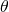has the limits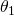and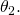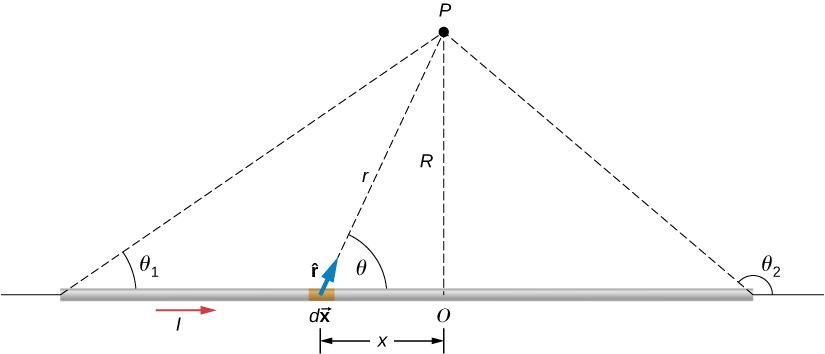Let’s begin by considering the magnetic field due to the current element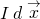located at the position x. Using the right-hand rule 1 from the previous chapter,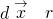points out of the page for any element along the wire. At point P, therefore, the magnetic fields due to all current elements have the same direction. This means that we can calculate the net field there by evaluating the scalar sum of the contributions of the elements. With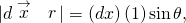we have from the Biot-Savart law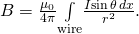The wire is symmetrical about point O, so we can set the limits of the integration from zero to infinity and double the answer, rather than integrate from negative infinity to positive infinity. Based on the picture and geometry, we can write expressions for r and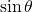in terms of x and R, namely: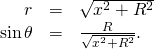Substituting these expressions into (Figure), the magnetic field integration becomes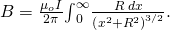Evaluating the integral yields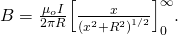Substituting the limits gives us the solution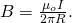The magnetic field lines of the infinite wire are circular and centered at the wire ((Figure)), and they are identical in every plane perpendicular to the wire. Since the field decreases with distance from the wire, the spacing of the field lines must increase correspondingly with distance. The direction of this magnetic field may be found with a second form of the right-hand rule (illustrated in (Figure)). If you hold the wire with your right hand so that your thumb points along the current, then your fingers wrap around the wire in the same sense as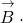Some magnetic field lines of an infinite wire. The direction of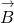can be found with a form of the right-hand rule.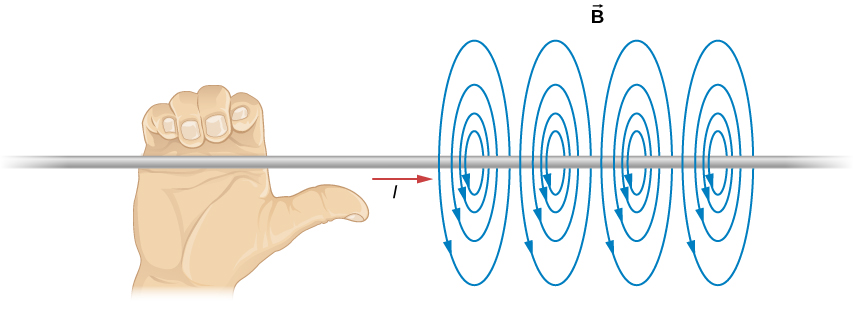The direction of the field lines can be observed experimentally by placing several small compass needles on a circle near the wire, as illustrated in (Figure). When there is no current in the wire, the needles align with Earth’s magnetic field. However, when a large current is sent through the wire, the compass needles all point tangent to the circle. Iron filings sprinkled on a horizontal surface also delineate the field lines, as shown in (Figure).

The shape of the magnetic field lines of a long wire can be seen using (a) small compass needles and (b) iron filings.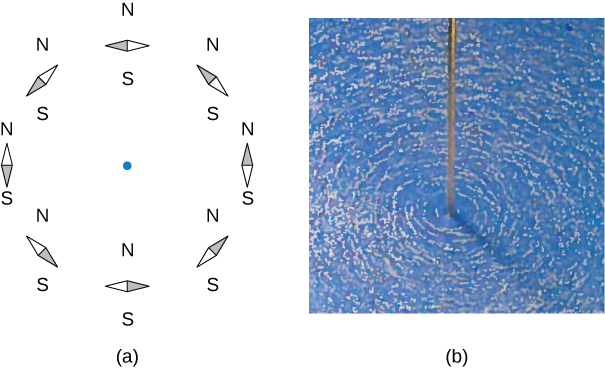Calculating Magnetic Field Due to Three Wires Three wires sit at the corners of a square, all carrying currents of 2 amps into the page as shown in (Figure). Calculate the magnitude of the magnetic field at the other corner of the square, point P, if the length of each side of the square is 1 cm.

Three wires have current flowing into the page. The magnetic field is determined at the fourth corner of the square.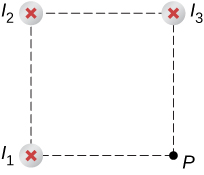Strategy The magnetic field due to each wire at the desired point is calculated. The diagonal distance is calculated using the Pythagorean theorem. Next, the direction of each magnetic field’s contribution is determined by drawing a circle centered at the point of the wire and out toward the desired point. The direction of the magnetic field contribution from that wire is tangential to the curve. Lastly, working with these vectors, the resultant is calculated.

Solution Wires 1 and 3 both have the same magnitude of magnetic field contribution at point P: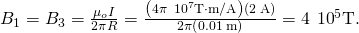Wire 2 has a longer distance and a magnetic field contribution at point P of: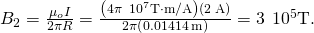The vectors for each of these magnetic field contributions are shown.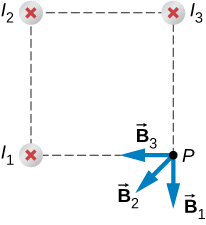The magnetic field in the x-direction has contributions from wire 3 and the x-component of wire 2: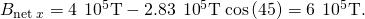The y-component is similarly the contributions from wire 1 and the y-component of wire 2: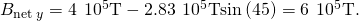Therefore, the net magnetic field is the resultant of these two components: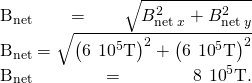Significance The geometry in this problem results in the magnetic field contributions in the x– and y-directions having the same magnitude. This is not necessarily the case if the currents were different values or if the wires were located in different positions. Regardless of the numerical results, working on the components of the vectors will yield the resulting magnetic field at the point in need.

Check Your Understanding Using (Figure), keeping the currents the same in wires 1 and 3, what should the current be in wire 2 to counteract the magnetic fields from wires 1 and 3 so that there is no net magnetic field at point P?

4 amps flowing out of the page

### Summary

• The strength of the magnetic field created by current in a long straight wire is given by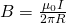(long straight wire) where I is the current, R is the shortest distance to the wire, and the constant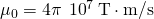is the permeability of free space.
• The direction of the magnetic field created by a long straight wire is given by right-hand rule 2 (RHR-2): Point the thumb of the right hand in the direction of current, and the fingers curl in the direction of the magnetic field loops created by it.

### Conceptual Questions

How would you orient two long, straight, current-carrying wires so that there is no net magnetic force between them? (Hint: What orientation would lead to one wire not experiencing a magnetic field from the other?)

You would make sure the currents flow perpendicular to one another.

### Problems

A typical current in a lightning bolt isA. Estimate the magnetic field 1 m from the bolt.

The magnitude of the magnetic field 50 cm from a long, thin, straight wire is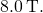What is the current through the long wire?

20 A

A transmission line strung 7.0 m above the ground carries a current of 500 A. What is the magnetic field on the ground directly below the wire? Compare your answer with the magnetic field of Earth.

A long, straight, horizontal wire carries a left-to-right current of 20 A. If the wire is placed in a uniform magnetic field of magnitude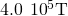that is directed vertically downward, what is the resultant magnitude of the magnetic field 20 cm above the wire? 20 cm below the wire?

Both answers have the magnitude of magnetic field of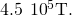The two long, parallel wires shown in the accompanying figure carry currents in the same direction. If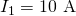and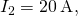what is the magnetic field at point P?

The accompanying figure shows two long, straight, horizontal wires that are parallel and a distance 2a apart. If both wires carry current I in the same direction, (a) what is the magnetic field at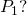(b)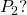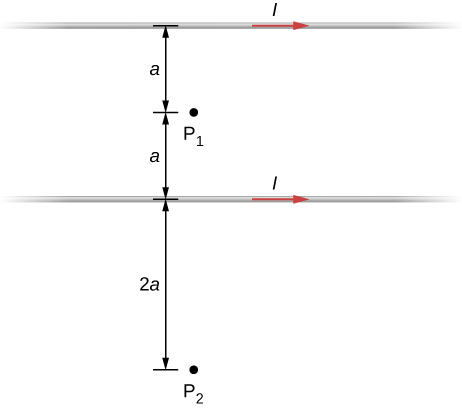At P1, the net magnetic field is zero. At P2,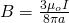into the page.

Repeat the calculations of the preceding problem with the direction of the current in the lower wire reversed.

Consider the area between the wires of the preceding problem. At what distance from the top wire is the net magnetic field a minimum? Assume that the currents are equal and flow in opposite directions.

The magnetic field is at a minimum at distance a from the top wire, or half-way between the wires.